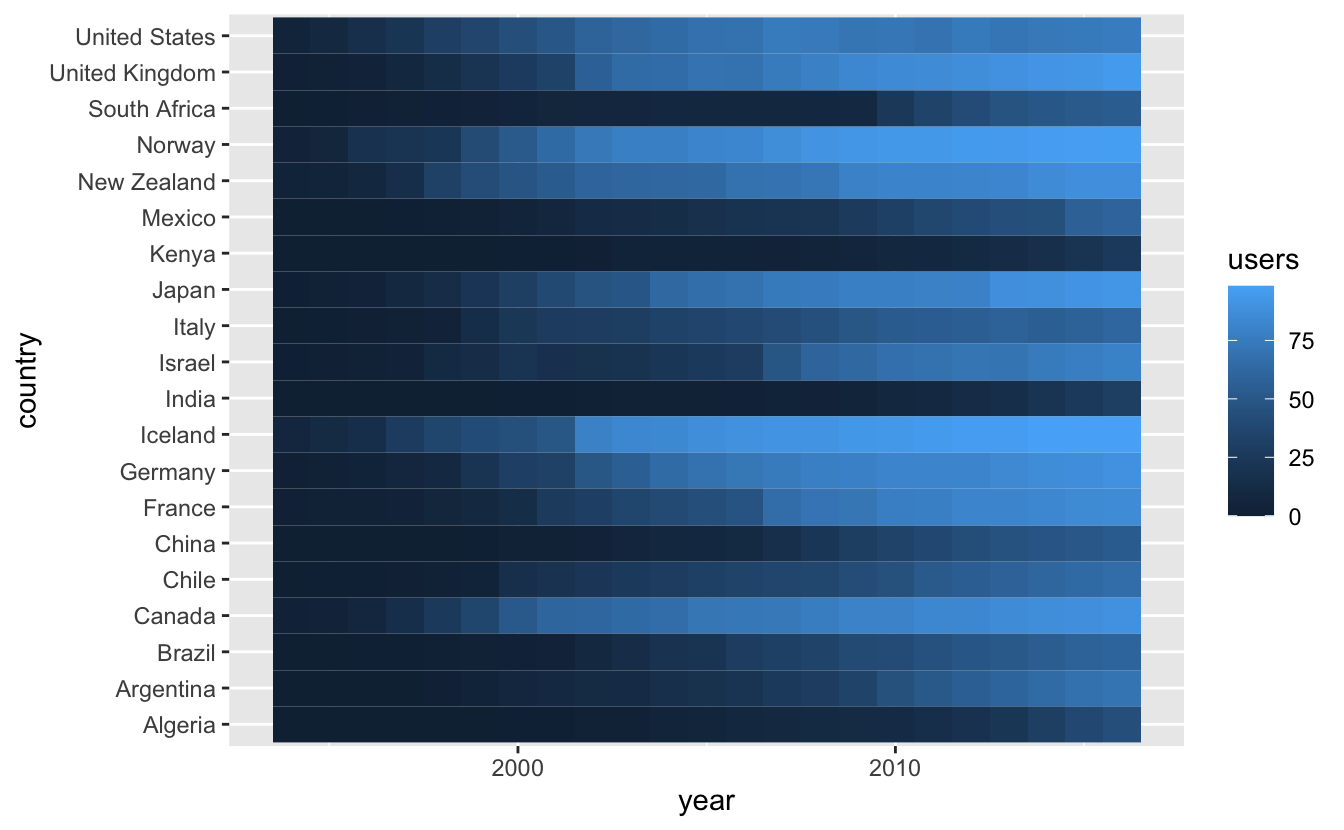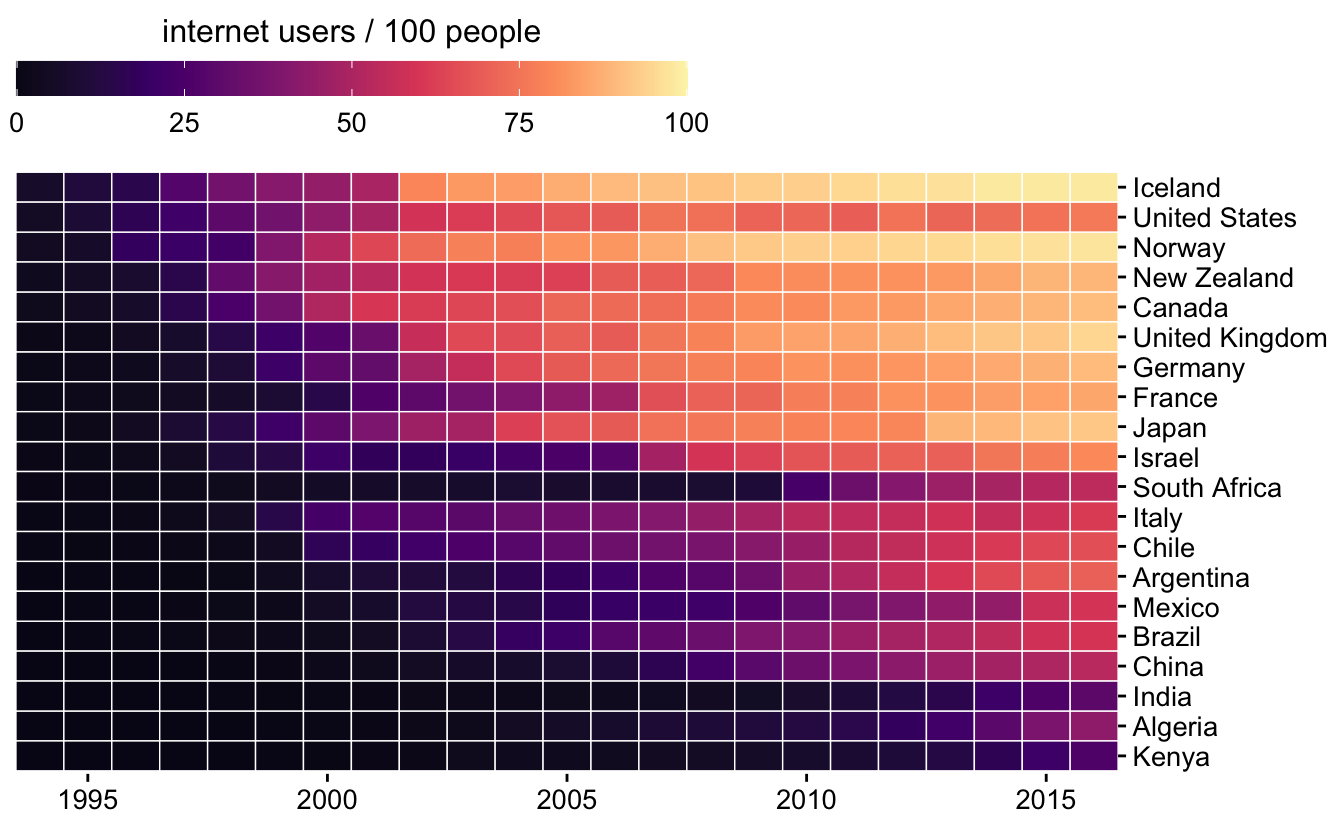Enter your name and EID here

This homework is due on March 22, 2021 at 11:00pm. Please submit as a pdf file on Canvas.

For both problems in this homework, we will work with the `internet` dataset. It contains the number of internet users over time for 20 select countries. Internet users are reported as percentages.

``````internet <- read_csv("https://wilkelab.org/SDS375/datasets/internet.csv")
internet``````
``````## # A tibble: 460 x 3
##    country         year    users
##    <chr>          <dbl>    <dbl>
##  1 Argentina       1994 0.0437
##  2 Brazil          1994 0.0377
##  3 Canada          1994 2.38
##  4 Chile           1994 0.141
##  5 China           1994 0.00117
##  6 Germany         1994 0.923
##  7 Algeria         1994 0.000361
##  8 France          1994 0.900
##  9 United Kingdom  1994 1.04
## 10 India           1994 0.00107
## # … with 450 more rows``````

Problem 1: (5 pts)

Take the following plot and make two modifications:

1. Put the countries into a meaningful order
2. Use scale and theme functions to improve the visual design of the plot

Grading rubric: 2 pts for ordering, 3 pts for visual design

``````# original plot as provided in homework
internet %>%
ggplot(aes(x = year, y = country, fill = users)) +
geom_tile()````````````# modified plot
internet %>%
mutate(
country = fct_reorder(country, users, min)
) %>%
ggplot(aes(x = year, y = country, fill = users)) +
geom_tile(color = "white", size = 0.25) +
scale_fill_viridis_c(
option = "A", begin = 0.05, end = 0.98,
limits = c(0, 100),
name = "internet users / 100 people",
guide = guide_colorbar(
direction = "horizontal",
title.position = "top",
barwidth = grid::unit(3.5, "in")
)
) +
scale_x_continuous(expand = c(0, 0), name = NULL) +
scale_y_discrete(name = NULL, position = "right") +
theme_half_open(12) +
theme(
axis.line = element_blank(),
legend.position = "top",
legend.title.align = 0.5
)``````Problem 2: (5 pts) Take the plot from the previous problem and make the following modifications:

1. Select a subset of 6 countries, using arbitrary criteria
2. Use `geom_line()` to show internet users over time, and use facets to show the different countries
3. Use a different ordering than you used in Problem 1.
4. Modify the visual design so it is appropriate for your new plot

Hint: To get started, see slides 33 to 43 in the class on getting things into the right order: https://wilkelab.org/SDS375/slides/getting-things-in-order.html#33

Grading rubric: 3 pts for making the right plot, 2 pts for visual design

``````countries <- c("Algeria", "Brazil", "Chile", "France", "Japan", "Norway")

internet %>%
filter(country %in% countries) %>%
mutate(country = fct_reorder(country, users, median)) %>%
ggplot(aes(x = year, y = users)) +
geom_line() +
scale_x_continuous(
limits = c(1993, 2017),
breaks = c(1995, 2000, 2005, 2010, 2015),
labels = c("1995",  "", "2005", "", "2015")
) +
scale_y_continuous(
name = "internet users",
breaks = c(0, 25, 50, 75, 100),
labels = c("0%", "25%", "50%", "75%", "100%"),
expand = c(0, 0),
limits = c(0, 100)
) +
facet_wrap(vars(country), scales = "free_x") +
theme_minimal_hgrid() +
theme(
panel.background = element_rect(fill = "gray95")
)``````